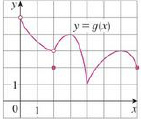Chapter 4.1, Problem 6E### Single Variable Calculus: Early Tr...

8th Edition
James Stewart
ISBN: 9781305270343

#### Solutions

Chapter
Section### Single Variable Calculus: Early Tr...

8th Edition
James Stewart
ISBN: 9781305270343
Textbook Problem

# Use the graph to state the absolute and local maximum and minimum values of the function.To determine

To state: The absolute maximum and minimum, local maximum and minimum of the given graph.

Explanation

The open dot in the graph indicates that the point is not included in the domain. Therefore, the point x=0 is not included in the domain. Thus, the maximum value does not occur at that point and hence there is no absolute maximum value.

Since g(4)g(x) for all x in domain, absolute minimum occurs at g(4)=1 .

Since g(3)g(x) for any x nearer to 3 and g(6)g(x) for any x nearer to 6

### Still sussing out bartleby?

Check out a sample textbook solution.

See a sample solution

#### The Solution to Your Study Problems

Bartleby provides explanations to thousands of textbook problems written by our experts, many with advanced degrees!

Get Started

#### Domain Find the domain of the expression. 10. 2t253t+6

Precalculus: Mathematics for Calculus (Standalone Book)

#### What is the lowest score in the following distribution?

Essentials of Statistics for The Behavioral Sciences (MindTap Course List)

#### Rationalize the numerator: x+yxy

Applied Calculus for the Managerial, Life, and Social Sciences: A Brief Approach

#### In Problems 19-44, factor completely. 39.

Mathematical Applications for the Management, Life, and Social Sciences

#### limx0+(1+x)1/x= a) e b) e c) 1e d) 1e

Study Guide for Stewart's Single Variable Calculus: Early Transcendentals, 8th

#### Define fraud and explain the safeguards that exist to prevent it.

Research Methods for the Behavioral Sciences (MindTap Course List)